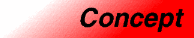# SequenceCategory: containers Component type: concept

## Description

A Sequence is a variable-sized Container whose elements are arranged in a strict linear order. It supports insertion and removal of elements.

## Associated types

None, except for those of ForwardContainer.

## Notation

 `X` A type that is a model of Sequence `a`, `b` Object of type `X` `T` The value type of `X` `t` Object of type `T` `p`, `q` Object of type `X::iterator` `n` Object of a type convertible to `X::size_type`

## Definitions

If `a` is a Sequence, then `p` is a valid iterator in a if it is a valid (nonsingular) iterator that is reachable from `a.begin()`.

If `a` is a Sequence, then `[p, q)` is a valid range in a if `p` and `q` are valid iterators in `a` and if `q` is reachable from `p`.

## Valid expressions

In addition to the expressions defined in ForwardContainer, the following expressions must be valid.

Name Expression Type requirements Return type
Fill constructor `X(n, t)`   `X`
Fill constructor `X a(n, t);`
Default fill constructor `X(n)` `T` is DefaultConstructible. `X`
Default fill constructor `X a(n);` `T` is DefaultConstructible.
Range constructor `X(i, j)` `i` and `j` are InputIterator whose value type is convertible to `T`  `X`
Range constructor `X a(i, j);` `i` and `j` are InputIterator whose value type is convertible to `T` 
Front `a.front()`   `reference` if `a` is mutable, `const_reference` otherwise.
Insert `a.insert(p, t)`   `X::iterator`
Fill insert `a.insert(p, n, t)` `a` is mutable `void`
Range insert `a.insert(p, i, j)` `i` and `j` are InputIterator whose value type is convertible to `T` . `a` is mutable `void`
Erase `a.erase(p)` `a` is mutable `iterator`
Range erase `a.erase(p,q)` `a` is mutable `iterator`
Clear `a.clear()` `a` is mutable `void`
Resize `a.resize(n, t)` `a` is mutable `void`
Resize `a.resize(n)` `a` is mutable `void`

## Expression semantics

Semantics of an expression is defined only where it is not defined in ForwardContainer, or where it differs.

Name Expression Precondition Semantics Postcondition
Fill constructor `X(n, t)` `n >= 0` Creates a sequence with `n` copies of `t` `size() == n`. Every element is a copy of `t`.
Fill constructor `X a(n, t);` `n >= 0` Creates a sequence with `n` copies of `t` `a.size() == n`. Every element of `a` is a copy of `t`.
Default fill constructor `X(n)` `n >= 0` Creates a sequence of `n` elements initialized to a default value. `size() == n`. Every element is a copy of `T()`.
Default fill constructor `X a(n, t);` `n >= 0` Creates a sequence with `n` elements initialized to a default value. `a.size() == n`. Every element of `a` is a copy of `T()`.
Default constructor `X a;` or `X()`   Equivalent to `X(0)`. `size() == 0`.
Range constructor `X(i, j)` `[i,j)` is a valid range. Creates a sequence that is a copy of the range `[i,j)` `size()` is equal to the distance from `i` to `j`. Each element is a copy of the corresponding element in the range `[i,j)`.
Range constructor `X a(i, j);` `[i,j)` is a valid range. Creates a sequence that is a copy of the range `[i,j)` `a.size()` is equal to the distance from `i` to `j`. Each element in `a` is a copy of the corresponding element in the range `[i,j)`.
Front `a.front()` `!a.empty()` Equivalent to `*(a.first())`
Insert `a.insert(p, t)` `p` is a valid iterator in `a`. `a.size() < a.max_size()` A copy of `t` is inserted before `p`.   `a.size()` is incremented by 1. `*(a.insert(p,t))` is a copy of `t`. The relative order of elements already in the sequence is unchanged.
Fill insert `a.insert(p, n, t)` `p` is a valid iterator in `a`. `n >= 0 && a.size() + n <= a.max_size()`. `n` copies of `t` are inserted before `p`.    `a.size()` is incremented by n. The relative order of elements already in the sequence is unchanged.
Range insert `a.insert(p, i, j)` `[i,j)` is a valid range. `a.size()` plus the distance from `i` to `j` does not exceed `a.max_size()`. Inserts a copy of the range `[i,j)` before `p`.    `a.size()` is incremented by the distance from `i` to `j`. The relative order of elements already in the sequence is unchanged.
Erase `a.erase(p)` `p` is a dereferenceable iterator in `a`. Destroys the element pointed to by `p` and removes it from `a`.  `a.size()` is decremented by 1. The relative order of the other elements in the sequence is unchanged. The return value is an iterator to the element immediately following the one that was erased.
Range erase `a.erase(p,q)` `[p,q)` is a valid range in `a`. Destroys the elements in the range `[p,q)` and removes them from `a`.  `a.size()` is decremented by the distance from `i` to `j`. The relative order of the other elements in the sequence is unchanged. The return value is an iterator to the element immediately following the ones that were erased.
Clear `a.clear()`   Equivalent to `a.erase(a.begin(), a.end())`
Resize `a.resize(n, t)` `n <= a.max_size()` Modifies the container so that it has exactly `n` elements, inserting elements at the end or erasing elements from the end if necessary. If any elements are inserted, they are copies of `t`. If `n > a.size()`, this expression is equivalent to `a.insert(a.end(), n - size(), t)`. If `n < a.size()`, it is equivalent to `a.erase(a.begin() + n, a.end())`. `a.size() == n`
Resize `a.resize(n)` `n <= a.max_size()` Equivalent to `a.resize(n, T())`. `a.size() == n`

## Complexity guarantees

The fill constructor, default fill constructor, and range constructor are linear.

Front is amortized constant time.

Fill insert, range insert, and range erase are linear.

The complexities of single-element insert and erase are sequence dependent.